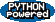PEAK-Rules/Indexing UserPreferences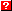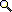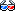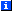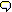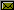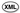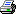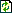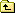You are not allowed to delete pages in this wiki!

# Decision Trees and Index Selection

One of the most efficient representations for executing a collection of rules is a decision tree expressed as code. This document describes the design (and tests the implementation) of a decision tree building subsystem and its indexing machinery. You do not need to read this unless you are extending these subsystems, e.g. to support outputting something other than bytecode, or to add specialized indexes, an alternate expression language, etc.

This document does not describe in detail how indexes are used to build executable decision trees (e.g. in bytecode or source code form), but focuses instead on the overall process of decision tree building, how indexes are selected to build individual nodes, and how these processes can be customized.

The algorithms presented here are based in part on the ones described by Craig Chambers and Weimin Chen in their 1999 paper on Efficient Multiple and Predicate Dispatching. We do, however, introduce an improved appraoch to managing inter-expression constraints. The new approach is simpler to explain and implement, while being more precise in what it constrains, as it more directly represents the actual constraints supplied by the input rules.

# Expression Selection

An "expression" is an object that is used to build individual decision tree nodes, using statistics about which rules accept what values for a particular argument expression. Different expression types are used to apply different kinds of criteria, such as isinstance() or issubclass() tests versus equality or other comparison tests. You can create new expression types to support new kinds of criteria.

For example, given rules with these criteria:

```isinstance(x,Foo) and y==BAR
isinstance(x,Baz) and y>0 and z/y<27
```

Three expressions would be required: one to handle isinstance() tests on x, one to handle comparison tests on y, and a third to handle comparison tests on z/y.

We would want the resulting decision tree to look something like this (ignoring inheritance and various other issues, of course):

```switch type(x):
case Foo:
if y==BAR:
...
case Baz:
if y>0:
if z/y<27:
...
```

The decision tree must meet two requirements: it must be correct, and it must be as efficient as possible. To be efficient, it must test highly selective expressions first. For example, it would be unwise to first test conditions that apply to only a small number of rules. In the above example, testing z/y<27 first would have been wasteful because only one of the two rules cares about the value of z/y.

To be correct, however, the tree must avoid reordering tests that are guarded by preconditions -- like y>0 and z/y<27, where the y>0 guards against division by zero. Even if it was highly selective, we can't use the z/y equality index for the root of the decision tree.

These two requirements of correctness and efficiency are met by managing inter-expression ordering constraints and selectivity statistics, as described in the sections below.

## Ordering Constraints

Inter-expression ordering constraints ensure that evaluation is not reordered in such a way as to test expressions before their guards. Support for tracking these guards is provided by the Ordering add-on of an engine:

```>>> from peak.rules.indexing import Ordering
>>> def f(): """An object to attach some orderings to"""
```

Ordering add-ons have two methods for managing inter-expression constraints: requires() and can_precede().

The requires() method adds a "constraint": a set of expressions that must all have been computed before the constrained expression can be used. Let's define a constraint for function f that says z/y can only be computed after x and y have been examined:

```>>> Ordering(f, "z/y").requires(["x", "y"])
```

We won't add constraints for tests on plain arguments like x and y themselves, because argument-only tests can be evaluated in any order. (Note, by the way, that although the examples here use strings, the actual keys or expression objects used can be any hashable object.)

An expression can't be used in a decision tree node unless at least one of its constraints is met. As a decision tree is built, the tree builder tracks what expressions haven't yet been used -- and if a required expression hasn't been used yet, any constraints that contain it are not met.

Initially, all of our indexes will be unused, but only x and y will be usable:

```>>> unused = ["x", "y", "z/y"]

>>> Ordering(f, "x").can_precede(unused)
True
>>> Ordering(f, "y").can_precede(unused)
True
>>> Ordering(f, "z/y").can_precede(unused)
False
```

Let's say that the tree builder decides to evaluate y, and then removes it from the unused set:

```>>> unused.remove("y")

>>> Ordering(f, "x").can_precede(unused)
True
>>> Ordering(f, "z/y").can_precede(unused)
False
```

Since x is still in the unused set, z/y still can't be used. We have to remove it also:

```>>> unused.remove("x")
>>> Ordering(f, "z/y").can_precede(unused)
True
```

### Multiple Constraints

You can add more than one ordering constraint for an expression, since the same expression may be used in different positions in different rules. For example, suppose we have the following criteria:

```E1 and E2 and E3 and E4
E5 and E3 and E2 and E6
```

E2 and E3 appear in two different places, resulting in the following ordering constraints for the first expression:

```>>> Ordering(f, "E2").requires(["E1"])  # E1 and E2 and E3 and E4
>>> Ordering(f, "E3").requires(["E1", "E2"])
>>> Ordering(f, "E4").requires(["E1", "E2", "E3"])
```

However, we can shortcut this repetitious process by using the define_ordering function, which automatically adds all the constraints implied by a given expression sequence:

```>>> from peak.rules.indexing import define_ordering
>>> define_ordering(f, ["E5","E3","E2","E6"])   # E5 and E3 and E2 and E6
```

All in all, E3 can be computed as long as either E1 and E2 or E5 have been computed. E2 can be computed as long as either E1 or E5 and E3 have been computed:

```>>> E2 = Ordering(f, "E2")

>>> E2.can_precede(["E1", "E2", "E3", "E4", "E5", "E6"])
False
>>> E2.can_precede(["E1", "E5"])
False
>>> E2.can_precede(["E5"])
True
>>> E2.can_precede(["E1"])
True
```

This is somewhat different from the Chambers & Chen approach, in that their approach reduces the above example to a constraint graph of the form:

```(E1 or E5) -> (E2 or E3) -> (E4 or E6)
```

Their approach is a little over-optimistic here, in that it assumes that "E5, E2" is a valid calculation sequence, even though this sequence appears nowhere in the input rules! The approach we use (which tracks the actual constraints provided by the rules) will allow any of these sequences to compute E2:

```E5, E3, E2
E1, E2
E5, E1, E2
E1, E5, E2
```

It does not overgeneralize from this to assume that "E5, E2" is valid, the way the Chambers & Chen approach does.

### Constraint Simplification

The active constraints of an index are maintained as a set of frozen sets:

```>>> from peak.rules.indexing import set, frozenset  # 2.3 compatibility

>>> E2.constraints == set([frozenset(["E1"]), frozenset(["E5", "E3"])])
True
```

Because constraints are effectively "or"ed together, adding a more restrictive constraint than an existing constraint is ignored:

```>>> E2.requires(["E5", "E3", "E4"])
>>> E2.constraints == set([frozenset(["E1"]), frozenset(["E5", "E3"])])
True
```

And if a less-restrictive constraint is added, it replaces any constraints that it's a subset of:

```>>> E2.requires(["E5"])
>>> E2.constraints == set([frozenset(["E1"]), frozenset(["E5"])])
True
```

And of course adding the same constraint more than once has no effect:

```>>> E4 = Ordering(f, "E4")
>>> E4.requires(["E1", "E2", "E3"])
>>> E4.requires(["E1", "E2", "E3"])
>>> E4.constraints == set([frozenset(["E1", "E2", "E3"])])
True
```

## Selectivity Statistics

To maximize efficiency, decision tree nodes should always be built using the "most selective" expression that is currently legal to evaluate.

Selectivity isn't an absolute measurement, however. It's based on the cases remaining to be distinguished at the current node. If none of the cases at a node care about a particular expression, that expression shouldn't be used. Likewise, if all of the rules care about an expression, but they are all expecting the same class or value, there may be better choices that would narrow down the applicable rules faster.

All of these conditions can be determined using two statistics: the number of branches that the expression would produce for the current node, and the average number of cases remaining on each of the branches. If none of the cases care about the expression, then each branch will still have the same number of rules as the current node does. Thus the average is N (the number of cases at the current node.)

If all of the cases care about the expression, but they all expect the same class or value, then there would be two branches: one empty, and one with N rules. Thus, its average is N/2: better than the case where none of the rules care, but it could be better still.

If each rule expects a different value for the expression, then there will be N branches, each of length 1, resulting in an average of about 1 -- an optimal choice.

Decision tree builders must therefore be able to compute an expression's selectivity, as we will see in the next section.

# Decision Tree Building

The TreeBuilder class implements the basic algorithm for transforming rules into a decision tree:

```>>> from peak.rules.indexing import TreeBuilder
```

A decision tree is built by taking a set of cases (action definitions), and a set of indexes that cover all expressions tested by those cases, using the build() method:

build(cases, exprs, memo)

Builds a decision tree to distinguish cases, using exprs. The "best" expression (based on legality of use and selectivity) is chosen and used to build a dispach node, with subnodes recursively constructed by calls to build() with the remaining cases and exprs. Leaf nodes are constructed whenever either the cases or expressions are exhausted along a particular path. memo must be a dictionary.

This method is memoized via memo, meaning that if it is called more than once with the same cases and indexes, it will return the same result each time. That is, the first invocation's return value is cached in memo, and returned by future calls using the same memo. This helps cut down on the total decision tree size by eliminating the redundancy that would otherwise occur when more than one path leads to the same remaining options.

Because of this, however, the input cases and exprs must be hashable, because the TreeBuilder will be using them as dictionary keys. Our examples here will use tuples of cases and indexes (so as to maintain their order when we display them), but immutable sets could also be used, as could integer "bit sets" or strings or anything else that's hashable. The build() method doesn't care about what the cases or indexes "mean"; it just passes them off to other methods for handling.

## Subclassing TreeBuilder

The TreeBuilder base class requires the following methods to be defined in a subclass, in order for the build() template method to work:

build_node(expr, cases, remaining_exprs, memo)
Build a decision node, using expr as the expression to dispatch on. cases are the actions to be distinguished, and remaining_exprs are the expressions that still need dispatch nodes. This method should call back to the build() method to obtain subnodes as needed, via e.g. self.build(subnode_cases, remaining_indexes, memo). This method should then return the switch node it has constructed.
build_leaf(cases, memo)

Build a leaf node for cases. Usually this means something like picking the most specific case, or producing a method combination for the cases. The return value should be the leaf node it has constructed.

For improved efficiency, most TreeBuilder subclasses may wish to cache these leaf nodes by their value in memo, to allow equivalent leaves to be shared even if they were produced by different sets of cases. Such caching should be done by this method, if applicable.

selectivity(expr, cases)

Estimate the selectivity of a decision tree node that subdivides cases using expr. The return value must be a tuple of the form (branches, total), where branches is the number of branches that the node would have, and total is the total number of rules applying on each branch. (In other words, the average number of rules on each branch is total/branches.)

If expr is not used by any of the cases, the returned total must be equal to branches * len(cases). If expr is used, however, the return statistics can be estimated rather than precisely computed, if it improves performance. (Selectivity estimation is done a lot during tree building, since each node must choose the "best" expression from all the currently-applicable expressions.)

cost(expr, remaining_exprs)
Estimate the cost of computing expr, given that remaining_exprs have not been computed yet. Lower costs are better than higher ones. The default implementation of this method just returns 1. Expression cost is only used as a tiebreaker when the selectivity of two candidate expressions is the same, however.

For our examples, we'll define some of these methods to build interior nodes as dictionaries, and leaf nodes as lists. We'll also print out what we're doing, to show that redundant nodes are cached. And, we'll compute selectivity in a slow but easy way: by building a branch table for the expressions.

But first, we'll need an expression class. For simplicity's sake, we'll use a string subclass that uses sequences of name-value pairs as rules, and creates branches for each value whose name equals the expression string:

```>>> class DemoExpr(str):
...     def branch_table(self, cases):
...         branches = {None: []}   # "none of the above" branch
...         for case in cases:
...             care = False
...             for name, value in case:
...                 if name==self:
...                     branches.setdefault(value, []).append(case)
...                     care = True
...             if not care:
...                 # "don't care" rules must be added to *every* branch
...                 for b in branches:
...                     branches[b].append(case)
...         return branches

>>> def r(**kw):
...     return tuple(kw.items())

>>> x = DemoExpr('x')

>>> x.branch_table([r(x=1), r(x=2)]) == {
...     None: [], 1: [(('x', 1),)], 2: [(('x', 2),)]
... }
True
```

Notice, by the way, that branch tables produced by this expression type contain a None key, to handle the case where the expression value doesn't match any of the rules. It isn't necessary that this key actually be None, and for many types of expression in PEAK-Rules, it can't be None. But the general idea of a "none-of-the-above" branch in tree nodes is nonetheless important.

(Note also that expression objects aren't required to have a branch_table() method; that's just an implementation detail of the demos in this document.)

## Computing Selectivity and Building Nodes

Now that we have our expression type and the ability to build simple branch tables, we can define our tree builder, which assumes that each "case" is a tuple of name-value pairs, and that each "expr" is a DemoExpr instance:

```>>> class DemoBuilder(TreeBuilder):
...
...     def build_node(self, expr, cases, remaining_exprs, memo):
...         enames = list(remaining_exprs)
...         enames.sort()
...         enames = ", ".join(enames)
...
...         print "building switch for", expr,
...         print "with", map(list,cases), "and (", enames, ")"
...
...         branches = expr.branch_table(cases).items()
...         branches.sort()
...         return dict(
...             [(key, self.build(tuple(values), remaining_exprs, memo))
...                 for key,values in branches]
...         )
...
...     def build_leaf(self, cases, memo):
...         print "building leaf node for", map(list,cases)
...         return map(list,cases)
...
...     def selectivity(engine, expr, cases):
...         branches = expr.branch_table(cases)
...         total = 0
...         for value, rules in branches.items():
...             total += len(rules)
...         return len(branches), total
```

Note, by the way, that this demo builder is very inefficient. A real builder would have other methods to allow cases to be added to it in advance for indexing purposes, and the selectivity and branch table calculations would be done by extracting a subset of precomputed index information. However, for this demo index, clarity and simplicity are more important than performance. In later sections, we'll look at more efficient ways to compute selectivity and build dispatch tables.

Here's a quick example to show how selectivity should be calculated for a few simple cases:

```>>> db = DemoBuilder()

>>> db.selectivity(x, [r(x=1), r(x=2)])  # 3 branches: 1, 2, None
(3, 2)
>>> db.selectivity(x, [r(x=1), r(x=1)])  # 2 branches: 1, None
(2, 2)
>>> db.selectivity(x, [])                # 1 branch: "None of the above"
(1, 0)
>>> db.selectivity(x, [r(y=42)])         # 1 branch: "None of the above"
(1, 1)
>>> db.selectivity(x, [r(x=1), r(y=1)])  # 2 branches: 1, None
(2, 3)
>>> db.selectivity(x, [r(y=2), r(z=1)])  # 1 branch: "None of the above"
(1, 2)
```

## Basic Tree-Building

Building nothing produces an empty leaf node:

```>>> DemoBuilder().build((), (), {})
building leaf node for []
[]
```

In fact, building anything with no remaining indexes produces a leaf node:

```>>> DemoBuilder().build((r(x=1),), (), {})
building leaf node for [[('x', 1)]]
[[('x', 1)]]
```

Any inapplicable indexes are ignored:

```>>> DemoBuilder().build((r(x=1),), (DemoExpr('q'),), {})
building leaf node for [[('x', 1)]]
[[('x', 1)]]
```

But applicable indexes are used:

```>>> DemoBuilder().build((r(x=1),), (DemoExpr('x'),), {}) == {
...     None: [], 1: [[('x', 1)]]
... }
building switch for x with [[('x', 1)]] and (  )
building leaf node for []
building leaf node for [[('x', 1)]]
True

>>> DemoBuilder().build((r(x=1),), (DemoExpr('x'), DemoExpr('q')), {}) == {
...     None: [], 1: [[('x', 1)]]
... }
building switch for x with [[('x', 1)]] and (  )
building leaf node for []
building leaf node for [[('x', 1)]]
True

>>> DemoBuilder().build((r(x=1),), (DemoExpr('q'), DemoExpr('x')), {}) == {
...     None: [], 1: [[('x', 1)]]
... }
building switch for x with [[('x', 1)]] and (  )
building leaf node for []
building leaf node for [[('x', 1)]]
True
```

As long as they have no constraints preventing them from being used:

```>>> def f(): pass
>>> x = DemoExpr('x')
>>> y = DemoExpr('y')
>>> db = DemoBuilder()
>>> Ordering(db, x).requires([y])
>>> db.build((r(x=1, y=2),), (x, y), {}) == {
...     None: [], 2: {None: [], 1: [[('y', 2), ('x', 1)]]}
... }
building switch for y with [[('y', 2), ('x', 1)]] and ( x )
building leaf node for []
building switch for x with [[('y', 2), ('x', 1)]] and (  )
building leaf node for [[('y', 2), ('x', 1)]]
True

>>> db.build((r(x=1, y=2),), (y, x), {}) == {
...     None: [], 2: {None: [], 1: [[('y', 2), ('x', 1)]]}
... }
building switch for y with [[('y', 2), ('x', 1)]] and ( x )
building leaf node for []
building switch for x with [[('y', 2), ('x', 1)]] and (  )
building leaf node for [[('y', 2), ('x', 1)]]
True
```

## Optimizations

If more than one index is applicable, the one with the best selectivity is chosen:

```>>> z = DemoExpr('z')
>>> rules = r(x=1,y=2,z=3), r(z=4)

>>> db.build(rules, (x,y,z), {}) == {   # doctest: +NORMALIZE_WHITESPACE
...     None: [],
...         3: {None: [],
...                2: {None: [],
...                       1: [[('y', 2), ('x', 1), ('z', 3)]]}},
...         4: [[('z', 4)]]
... }
building switch for z with
[[('y', 2), ('x', 1), ('z', 3)], [('z', 4)]] and ( x, y )
building leaf node for []
building switch for y with [[('y', 2), ('x', 1), ('z', 3)]] and ( x )
building switch for x with [[('y', 2), ('x', 1), ('z', 3)]] and (  )
building leaf node for [[('y', 2), ('x', 1), ('z', 3)]]
building leaf node for [[('z', 4)]]
True

>>> db.build(rules, (z,y,x), {}) == { # doctest: +NORMALIZE_WHITESPACE
...     None: [],
...        3: {None: [],
...               2: {None: [],
...                      1: [[('y', 2), ('x', 1), ('z', 3)]]}},
...        4: [[('z', 4)]]
... }
building switch for z with
[[('y', 2), ('x', 1), ('z', 3)], [('z', 4)]] and ( x, y )
building leaf node for []
building switch for y with [[('y', 2), ('x', 1), ('z', 3)]] and ( x )
building switch for x with [[('y', 2), ('x', 1), ('z', 3)]] and (  )
building leaf node for [[('y', 2), ('x', 1), ('z', 3)]]
building leaf node for [[('z', 4)]]
True
```

If an index is skipped due to a constraint, its selectivity should still be checked if its constraints go away (due to the blocking index being found inapplicable):

```>>> Ordering(db, z).requires([y])       # z now must have y checked first
>>> rules = r(x=1,z=3), r(z=4)  # but y isn't used by the rules

>>> db.build(rules, (x,y,z), {}) == {   # so z is the most-selective index
...     None: [], 3: {None: [], 1: [[('x', 1), ('z', 3)]]}, 4: [[('z', 4)]]
... }
building switch for z with [[('x', 1), ('z', 3)], [('z', 4)]] and ( x )
building leaf node for []
building switch for x with [[('x', 1), ('z', 3)]] and (  )
building leaf node for [[('x', 1), ('z', 3)]]
building leaf node for [[('z', 4)]]
True

>>> db.build(rules, (z,y,x), {}) == {
...     None: [], 3: {None: [], 1: [[('x', 1), ('z', 3)]]}, 4: [[('z', 4)]]
... } # try them in another order
building switch for z with [[('x', 1), ('z', 3)], [('z', 4)]] and ( x )
building leaf node for []
building switch for x with [[('x', 1), ('z', 3)]] and (  )
building leaf node for [[('x', 1), ('z', 3)]]
building leaf node for [[('z', 4)]]
True
```

The above examples whose results contain more than one [] leaf node show that leaf nodes for the same cases are being cached, but let's also show that non-leaf nodes are similarly shared and cached:

```>>> rules = (('x',1),('x',2),('y',1),('y',2)),
>>> tree = db.build(rules, (x,y,), {})
building switch for y
with [[('x', 1), ('x', 2), ('y', 1), ('y', 2)]] and ( x )
building leaf node for []
building switch for x
with [[('x', 1), ('x', 2), ('y', 1), ('y', 2)]] and (  )
building leaf node for [[('x', 1), ('x', 2), ('y', 1), ('y', 2)]]

>>> tree == {
...     None: [],
...        1: {None: [],
...               1: [[('x', 1), ('x', 2), ('y', 1), ('y', 2)]],
...               2: [[('x', 1), ('x', 2), ('y', 1), ('y', 2)]]},
...        2: {None: [],
...               1: [[('x', 1), ('x', 2), ('y', 1), ('y', 2)]],
...               2: [[('x', 1), ('x', 2), ('y', 1), ('y', 2)]]}
... }
True

>>> tree is tree
True

>>> tree is tree
True

>>> tree[None] is tree[None]
True
```

As you can see, the redundant leaf nodes and intermediate nodes are shared due to the caching, and the log shows that only two intermediate nodes and two leaf nodes were even created in the first place.

# Bitmap/Set Indexes

The basic indexes used by PEAK-Rules use a combination of bitmaps and sets to represent criteria as ranges or regions in a (1-dimensional) logical space. Points that represent region edges are known as "seeds".

In the simplest possible indexes, a bitmap of applicable rules (cases, actually) is kept for each seed. (For equality or identity testing, this is all that's required, but more complex criteria things get a bit more involved.)

For simple generic functions with 31 or fewer cases, a single integer is sufficient to represent any set of cases. For more complex generic functions, Python's long integer arithmetic performance scales reasonably well, even up to many hundreds of bits (cases). Plus, both integers and longs can be used as dictionary keys, and thus work well with the TreeBuilder class (which needs to cache dispatch nodes for sets of applicable cases).

## Bitmap Operations

To work with bitsets, we need to be able to convert an integer sequence to a bitmap (integer or long integer), and vice versa:

```>>> from peak.rules.indexing import to_bits, from_bits

>>> odds = to_bits([9,11,13,1,3,5,7,15])
>>> print hex(odds)
0xaaaa

>>> list(from_bits(odds))
[1, 3, 5, 7, 9, 11, 13, 15]
```

And to handle sets with more than 31 bits, we need these operations to handle long integers:

```>>> seven_long = to_bits([32,33,34])
>>> print hex(seven_long)
0x700000000L

>>> list(from_bits(seven_long))
[32, 33, 34]
```

## The BitmapIndex Add-on

The BitmapIndex add-on base class provides basic support for indexing cases identified by sequential integers. Indexes are keyed by expression and attach to an "engine", which can be any object that supports add-ons.

The BitmapIndex add-on provides these methods and attributes:

Given an integer case_id, update the index by calling the .add_criterion() method on criterion and caching the result (so that multiple cases using the same criterion share the same seeds).
add_criterion(criterion) (abstract method: must be defined in a subclass)

The .add_criterion() method must return a set or sequence of the "applicable seeds" for which the criterion holds true. It must also ensure that the .all_seeds index is up to date, usually by calling the .include() and .exclude() or .add_seed() methods.

The set or sequence returned by this method is only used for its len() as a basis for computing selectivity; its contents aren't actually used by the BitmapIndex base class. Thus, this method can return a specialized dynamic object that simply computes an appropriate length, and optionally updates the all_seeds sets when it notice new seeds there (e.g. due to reseed() or the addition of new cases and criteria).

include(seed, criterion)

Add criterion to the "inclusions" for seed. You should call this method in .add_criterion() for each seed that the criterion applies to.

Note that there is no requirement that the criterion used here must be limited to criteria that were passed to .add_criterion() -- that method is allowed to pre-calculate inclusions for related criteria that haven't been seen yet. For example, TypeIndex precalculates many inclusions and exclusions for the base classes of criteria it encounters.

exclude(seed, criterion)

Add criterion to the "exclusions" for seed. Like .include(), this is usually called from within .add_criterion(), and may be called on any criterion, whether or not it was passed to .add_criterion().

Simple indexes will probably have no use for this method, as "exclusions" are typically only useful for negated criteria. For example, an is not condition is false for all seeds but one, so it's not efficient to try to update the inclusions for every seed to list every is not criterion. Instead, it's better to record an exclusion for the is not criterion's seed, and an inclusion for the criterion to a "default" seed, so that by default, the criterion will apply.

selectivity(case_id_list)
Given a sorted sequence of integer case ids, return the selectivity statistics of the index for those cases. The default implementation simply sums the len() of the values returned by the appropriate .add_criterion() calls. It can be overridden in a subclass to implement more sophisticated calculation strategies.
seed_bits(cases)
Given a bitmap of cases, return a tuple (dontcares, seedmap), where dontcares is a bitset of "don't care" cases (i.e., cases that must be included in every child node), and seedmap is a dictionary mapping seeds to (include, exclude) bitset pairs.
reseed(criterion)
Handle a reseed request. By default, this is a synonym for .add_criterion(criterion), but if necessary it can be overridden in a subclass to handle reseeds differently.
expanded_sets()
Return a list of (seed, (inc, exc)) tuples, where inc and exc are integer case lists. This method is for debugging and testing only.
all_seeds
A dictionary containing information about all seeds seen by the index. The keys are the seeds, and the values are (include,exclude) tuples of sets of criteria, indicating which criteria include or exclude that key, respectively. Dynamic criteria should update this dictionary when new seeds are discovered (e.g. via reseed() or new cases being added).
criteria_bits
A dictionary mapping each known criterion to a bitset representing the cases that use that criterion.
criteria_seeds
A dictionary that caches the result of the index's calls to the .add_criterion() method.
case_seeds
A list mapping case ids to the values returned by .add_criterion() for the corresponding criterion. Entries for case ids that were not assigned criteria via .add_case() are filled with self.null.
null
The value used to pad skipped entries in the .case_seeds list. By default, this is the same object as self.all_seeds, so that for selectivity-calculation purposes, the case is treated as applying for all seeds. (You may change this in a subclass if you are also overriding the selectivity() method.)
known_cases
A bitset representing the case ids of all cases added to the index using the add_case() method.
extra
An empty dictionary, made available for the use of .add_criterion() algorithms that may require additional storage for dependencies or intermediate indexes.
match
A single-element sequence that can be used as a default return value from .add_criterion(). If your .add_criterion() method is going to return a set or sequence containing only one element (and it won't be changed later), you should return self.match instead of making a new sequence. This can save a lot of memory.

For our first demo, we'll assume we're indexing a simple equality condition, so every criterion will seed only to itself. We'll create a simple criterion type, and a suitable BitmapIndex subclass:

```>>> from peak.rules.indexing import BitmapIndex
>>> from peak.util.decorators import struct
>>> from peak.rules import when

>>> class DemoEngine: pass

>>> def find(val):
...     """A structure type used as a criterion"""
...     return val,
>>> find = struct()(find)

>>> class DemoIndex(BitmapIndex):
...     def add_criterion(self, criterion):
...         print "computing seeds for", criterion
...         self.include(criterion.val, criterion)
...         return [criterion.val]
```

By the way, the BitmapIndex constructor is an abstract factory: it can automatically create a subclass of the appropriate type, for the given engine and expression:

```>>> eng = DemoEngine()
>>> ind = BitmapIndex(eng, "some expression")
Traceback (most recent call last):
...
NoApplicableMethods: ((<...DemoEngine ...>, 'some expression'), {})
```

Well, it will as long as you register an appropriate method for the bitmap_index_type generic function:

```>>> from peak.rules.indexing import bitmap_index_type
>>> f = when(bitmap_index_type, (DemoEngine, str))(
...     lambda engine, expr: DemoIndex
... )
```

Now we should be able to get the right kind of index, just by calling BitmapIndex():

```>>> BitmapIndex(eng, "some expression")
<DemoIndex object at ...>
```

We also could have explicitly used DemoIndex, of course. Once created, the same instance will be reused for each call to either the specific constructor or BitmapIndex.

To begin with, our index's selectivity is 0/0 because there are no seeds (and therefore no branches):

```>>> ind = DemoIndex(eng, "some expression")

>>> ind.selectivity([])
(0, 0)

>>> ind.all_seeds
{}

>>> ind.criteria_seeds
{}

>>> list(from_bits(ind.known_cases))
[]
```

By adding a case, we'll add a criterion (and therefore a seed), which gets cached:

```>>> ind.add_case(0, find("x"))
computing seeds for find('x',)

>>> ind.criteria_seeds
{find('x',): ['x']}

>>> ind.criteria_bits
{find('x',): 1}
```

Now, selectivity will always be at least 1/0, because there's one possible branch:

```>>> ind.selectivity([])
(1, 0)
>>> ind.selectivity()
(1, 1)
>>> ind.selectivity()
(1, 1)
>>> ind.selectivity([1,2])
(1, 2)
```

And the seed bitmaps reflect this:

```>>> list(from_bits(ind.known_cases))


>>> ind.seed_bits(ind.known_cases)
(0, {'x': (1, 0)})

>>> dict(ind.expanded_sets())   # expanded form of seed_bits(known_cases)
{'x': [, []]}
```

If we add another case with the same criterion, the number of branches will stay the same. Notice also, that the seeds for a previously-seen criterion are not recalculated:

```>>> ind.add_case(1, find("x"))

>>> ind.criteria_bits   # criterion 'x' was used for cases 0 and 1
{find('x',): 3}

>>> dict(ind.expanded_sets())
{'x': [[0, 1], []]}

>>> list(from_bits(ind.known_cases))
[0, 1]

>>> ind.selectivity([])
(1, 0)
>>> ind.selectivity()
(1, 1)
>>> ind.selectivity()
(1, 1)
>>> ind.selectivity([1,2])
(1, 2)
```

However, if we add a new case with a new criterion, its seeds are computed, and the number of branches increases:

```>>> ind.add_case(2, find("y"))
computing seeds for find('y',)

>>> dict(ind.expanded_sets())
{'y': [, []], 'x': [[0, 1], []]}

>>> list(from_bits(ind.known_cases))
[0, 1, 2]

>>> ind.selectivity([])
(2, 0)
>>> ind.selectivity()
(2, 1)
>>> ind.selectivity()
(2, 1)
>>> ind.selectivity([1,2])
(2, 2)
```

# Indexing Criteria

All of the criterion objects provided by peak.rules.criteria have BitmapIndex subclasses suitable for indexing them:

```>>> from peak.rules.criteria import Value, Range, IsObject, Class, Conjunction
```

To demonstrate them, we'll use a dummy engine object:

```>>> class Engine: pass
>>> eng = Engine()
```

## Object Identity

The IsObject criterion type implements indexing for the is and is not operators. IsObject(x) represents is x, and IsObject(x, False) represents is not x. The bitmap index seeds for IsObject objects are the id() values of the target objects, or None to represent the "none of the above" cases:

```>>> from peak.rules.indexing import PointerIndex

>>> p = object()
>>> ppeq = IsObject(p)
>>> ppne = IsObject(p, False)

>>> ind = PointerIndex(eng, "x")

>>> dict(ind.expanded_sets())
{...: [, []], None: [[], ]}
```

The selectivity of an is criterion is 1:

```>>> ind.selectivity()
(2, 1)
```

And for an is not criterion, it's always one less than the total number of seeds currently in the index (because an is not criterion is true for every possible branch except its target):

```>>> ind.add_case(1, ppne)

>>> dict(ind.expanded_sets()) == {id(p): [, ], None: [, ]}
True

>>> ind.selectivity()
(2, 1)

>>> ind.selectivity([0,1])
(2, 2)

>>> q = object()

>>> ind.selectivity()    # now it's (3,2) instead of (2,1) or (3,1)
(3, 2)

>>> ind.selectivity()    # 'is' pointers are always 1
(3, 1)

>>> dict(ind.expanded_sets()) == {
...     None: [, [0, 2]], id(p): [, ], id(q): [[1, 2], []],
... }
True

>>> from peak.rules.core import intersect
>>> ind.add_case(3, intersect(ppne, IsObject(q,False)))

>>> dict(ind.expanded_sets()) == {
...     None: [[1, 3], [0, 2]], id(p): [, [1, 3]], id(q): [[1, 2], ],
... }
True

>>> r = object()

>>> dict(ind.expanded_sets()) == {
...     None: [[1, 3], [0, 2, 4]], id(p): [, [1, 3]],
...     id(q): [[1, 2], ], id(r): [[1, 3, 4], []]
... }
True
```

## Ranges and Value Comparisons

### Range Objects

The Range() criterion type represents an inequality such as lo < x < hi or x >= lo. (For more details on their semantics, see Criteria.txt).

The bitmap index seeds for Range objects are edge tuples, and the selectivity of a Range is the distance between the low and high edges in a sorted list of all the index's seeds:

```>>> from peak.rules.indexing import RangeIndex

>>> ind = RangeIndex(eng, "y")

>>> r = Range((1,-1), (23,1))

>>> ind.selectivity()
(2, 1)

>>> from peak.rules.criteria import sorted
>>> sorted(ind.expanded_sets())
[((1, -1), [, []]), ((23, 1), [[], ])]

>>> ind.add_case(1, Range((5,-1), (20,1)))
>>> ind.selectivity()
(4, 1)

>>> sorted(ind.expanded_sets())
[((1, -1), [, []]), ((5, -1), [, []]),
((20, 1), [[], ]), ((23, 1), [[], ])]

>>> ind.add_case(2, Range((7,-1), (24,1)))
>>> ind.selectivity()
(6, 3)

>>> sorted(ind.expanded_sets())
[((1, -1), [, []]), ((5, -1), [, []]),
((7, -1), [, []]), ((20, 1), [[], ]), ((23, 1), [[], ]),
((24, 1), [[], ])]

>>> ind.add_case(3, Range((7,-1), (7,1)))
>>> sorted(ind.expanded_sets())
[((1, -1), [, []]),
((5, -1), [, []]), ((7, -1), [[2, 3], []]), ((7, 1), [[], ]),
((20, 1), [[], ]), ((23, 1), [[], ]), ((24, 1), [[], ])]

>>> ind.selectivity()
(7, 5)
>>> ind.selectivity()
(7, 3)
>>> ind.selectivity()
(7, 4)
>>> ind.selectivity()
(7, 1)
```

### Value Objects

Value objects are used to represent == and != comparisons. Value(x) represents ==x and Value(x, False) represents !=x. The bitmap index seeds for Value objects are (value, 0) tuples, which fall between the "below" and "above" tuples of any Range objects in the same index. And the selectivity of a Value is either 1 or the number of seeds in the index, minus one:

```   >>> ind.add_case(4, Value(7, False))
>>> ind.selectivity()
(9, 8)

>>> sorted(ind.expanded_sets())
[((Min, -1), [, []]), ((1, -1), [, []]),
((5, -1), [, []]), ((7, -1), [[2, 3], []]), ((7, 0), [[], ]),
((7, 1), [[], ]), ((20, 1), [[], ]), ((23, 1), [[], ]),
((24, 1), [[], ])]

>>> ind.selectivity()
(9, 1)

>>> sorted(ind.expanded_sets())
[((Min, -1), [, ]), ((1, -1), [, []]),
((5, -1), [, []]), ((7, -1), [[2, 3], []]), ((7, 0), [, ]),
((7, 1), [[], ]), ((20, 1), [[], ]), ((23, 1), [[], ]),
((24, 1), [[], ])]

Notice that the seeds for a ``Value`` always include either an inclusion or
exclusion for ``(Min, -1)``, as this
```

### Value Map Generation

```>>> from peak.rules.indexing import split_ranges
>>> from peak.util.extremes import Min, Max
```
```>>> def dump_ranges(ind, cases):
...     exact, ranges = split_ranges(*ind.seed_bits(cases))
...     for k in exact.keys():
...         exact[k] = list(from_bits(exact[k]))
...     for n, (k, v) in enumerate(ranges):
...         ranges[n] = k, list(from_bits(v))
...     return exact, ranges
```
```>>> dump_ranges(ind, ind.known_cases) == (
...     {1: [0, 4], 5: [0, 1, 4], 7: [0, 1, 2, 3, 5], 20: [0, 1, 2, 4],
...      23: [0, 2, 4], 24: [2, 4]},
...     [((Min, 1), ), ((1, 5), [0, 4]), ((5, 7), [0, 1, 4]),
...      ((7, 20), [0, 1, 2, 4]), ((20, 23), [0, 2, 4]), ((23, 24), [2, 4]),
...      ((24, Max), )]
... )
True
```
```>>> ind = RangeIndex(eng, 'q')
>>> dump_ranges(ind, ind.known_cases) == ({}, [((Min, Max), [])])
True
```
```>>> ind.add_case(0, Value(19))
>>> dump_ranges(ind, ind.known_cases) == (
...     {Min: [], 19: }, [((Min, Max), [])]
... )
True
```
```>>> ind.add_case(1, Value(23))
>>> dump_ranges(ind, ind.known_cases) == (
...     {Min: [], 19: , 23: }, [((Min, Max), [])]
... )
True
```
```>>> ind.add_case(2, Value(23, False))
>>> dump_ranges(ind, ind.known_cases) == (
...     {19: [0, 2], 23: }, [((Min, Max), )]
... )
True
```
```>>> ind.add_case(3, Range(lo=(57,1)))
>>> dump_ranges(ind, ind.known_cases) == (
...     {57: , 19: [0, 2], 23: },
...     [((Min, 57), ), ((57, Max), [2, 3])]
... )
True
```
```>>> ind.add_case(4, Range(lo=(57,-1)))
>>> dump_ranges(ind, ind.known_cases) == (
...     {57: [2, 4], 19: [0, 2], 23: },
...     [((Min, 57), ), ((57, Max), [2, 3, 4])]
... )
True
```

## Single Classes

Class objects represent issubclass() or isinstance() tests. Class(x) is a instance/subclass match, while Class(x, False) is a non-match:

```>>> from peak.rules.indexing import TypeIndex

>>> ind = TypeIndex(eng, 'by class')
>>> ind.selectivity()
(2, 1)
>>> dict(ind.expanded_sets()) == {
...     int: [, []], object: [[], []]
... }
True

>>> class myint(int): pass
>>> ind.selectivity()
(3, 1)
>>> dict(ind.expanded_sets()) == {
...     int: [, []], object: [[], []], myint: [[0, 1], []]
... }
True

>>> ind.selectivity()
(3, 2)

>>> ind.add_case(2, Class(int, False))
>>> ind.selectivity()
(3, 1)
>>> dict(ind.expanded_sets()) == {
...     int: [, []], object: [, []], myint: [[0, 1], []]
... }
True

>>> class other(object): pass
>>> ind.selectivity()
(4, 1)
>>> ind.selectivity()
(4, 2)

>>> dict(ind.expanded_sets()) == {
...     int: [, []], object: [, []], other: [[2, 3], []],
...     myint: [[0, 1], []]
... }
True
```

## Multiple Classes

Conjunction objects can hold sets of 2 or more Class criteria, and represent the "and" of those criteria. This is the most complex type of criteria to index, because there's no easy way to incrementally update a set intersection:

```>>> class a(object): pass
>>> class b(object): pass
>>> class c(a,b): pass
>>> class d(b): pass
>>> class e(a,d): pass
>>> class f(e, c): pass
>>> class g(c): pass

>>> ind = TypeIndex(eng, 'classes')

>>> ind.add_case(0, Conjunction([Class(a), Class(b)]))
>>> ind.selectivity()
(3, 0)
>>> dict(ind.expanded_sets()) == {
...     a: [[], []], b: [[], []], object: [[], []]
... }
True

>>> ind.selectivity()    # c
(4, 1)
>>> ind.selectivity()
(4, 1)
>>> dict(ind.expanded_sets()) == {
...     a: [[], []], b: [[], []], c: [[0, 1], []], object: [[], []]
... }
True

>>> ind.add_case(2, Conjunction([Class(a), Class(b), Class(c, False)]))
>>> ind.selectivity()
(4, 0)

>>> dict(ind.expanded_sets()) == {
...     a: [[], []], b: [[], []], c: [[0, 1], []], object: [[], []]
... }
True

>>> ind.selectivity()    # c, e
(5, 2)
>>> ind.selectivity()
(5, 1)
>>> ind.selectivity()
(5, 1)
>>> dict(ind.expanded_sets()) == {
...     a: [[], []], b: [[], []], c: [[0, 1], []], object: [[], []],
...     e: [[0, 2, 3], []]
... }
True

>>> ind.selectivity()    # c, e, f
(6, 3)
>>> ind.selectivity()
(6, 1)
>>> dict(ind.expanded_sets()) == {
...     a: [[], []], b: [[], []], c: [[0, 1], []], object: [[], []],
...     e: [[0, 2, 3], []], f: [[0, 1, 3, 4], []]
... }
True

>>> ind.selectivity()    # c, e, f, g
(7, 4)
>>> ind.selectivity()    # still just 'e'
(7, 1)

>>> Conjunction([Class(d, False), Class(e, False)])
Class(<class 'd'>, False)

>>> ind.add_case(6, Conjunction([Class(d, False), Class(e, False)]))
>>> ind.selectivity()    # all but d, e, f
(8, 5)
>>> dict(ind.expanded_sets()) == {
...     a: [, []], b: [, []], c: [[0, 1, 6], []], object: [, []],
...     d: [[], []], e: [[0, 2, 3], []], f: [[0, 1, 3, 4], []],
...     g: [[0, 1, 5, 6], []],
... }
True
```

## Reseeding

Due to the nature of multiple inheritance, it's sometimes necessary to re-seed an index by adding a new criterion, recomputing selectivity, and then getting new seed bits. Let's test an example by making a new class that inherits from a and b:

```>>> class x(a,b): pass
```

This class isn't in the index, because we just created it. If it were only inheriting from one class, we could safely assume that a lookup of the base class would work just as well as looking up the actual class. However, since it inherits from more than one class, we don't know if there are multi-class criteria that might apply to it. (And in fact there are: cases 0 and 2 in our index apply to classes that inherit from both a and b.)

So, to update the index, we use the reseed() method:

```>>> ind.reseed(Class(x))
```

It takes a criterion and an optional bitset representing the cases for which a new seed_bits map should be generated. It updates the index by checking the len() of every "applicable seeds" set, after adding the criterion to the appropriate caches. The result is that the index now includes correct information for the new x class:

```>>> dict(ind.expanded_sets()) == {
...     a: [, []], b: [, []], c: [[0, 1, 6], []], object: [, []],
...     d: [[], []], e: [[0, 2, 3], []], f: [[0, 1, 3, 4], []],
...     g: [[0, 1, 5, 6], []], x: [[0, 2, 6], []]
... }
True
```

## Exact Types

Finally, let's try out some istype() and mixed class/classes/istype criteria, to make sure they interoperate:

```>>> from peak.rules import istype

>>> ind = TypeIndex(eng, 'types')
>>> ind.selectivity()
(2, 1)

>>> ind.add_case(1, istype(b, False))
>>> ind.selectivity()
(3, 2)

>>> ind.selectivity([0,1])
(3, 3)

>>> ind.selectivity()
(3, 1)

>>> ind.selectivity([0,1,2])
(3, 4)

>>> dict(ind.expanded_sets()) == {
...     a:[[0, 1, 2], []],
...     b:[[], []],
...     object:[, []]
... }
True

>>> ind.add_case(3, Class(a, False))
>>> ind.selectivity()
(3, 2)

>>> ind.selectivity([0,1,2,3])
(3, 6)

>>> dict(ind.expanded_sets()) == {
...     a:[[0, 1, 2], []],
...     b:[, []],
...     object:[[1, 3], []]
... }
True

>>> ind.add_case(4, Conjunction([Class(a), istype(c, False)]))
>>> ind.selectivity()
(4, 1)

>>> dict(ind.expanded_sets()) == {
...     a:[[0, 1, 2, 4], []],
...     b:[, []],
...     c:[[1, 2], []],
...     object:[[1, 3], []]
... }
True

>>> ind.reseed(Class(e))

>>> dict(ind.expanded_sets()) == {
...     a:[[0, 1, 2, 4], []],
...     b:[, []],
...     c:[[1, 2], []],
...     e:[[1, 2, 4], []],
...     object:[[1, 3], []]
... }
True

```

## Truth

TruthIndex treats boolean criteria as logical truth:

```>>> from peak.rules.indexing import TruthIndex

>>> ind = TruthIndex(eng, "z")